# 大整数乘法中的分治思想（TOOM-COOK的一种使用方法）

算法分析与设计学习中，接触到一道大整数乘法问题，分享出来，原题目如下：


X = | A| B | ， Y = | C| D|
A，B，C，D均为n/2位，求XY的问题可以转换为：

	X * Y = （A * 2^（n/2） +B ）  *（C * 2^（n/2） +D ）
= AC * 2^n + (AD + BC) * 2^（n/2）+BD



U = （A + B)(C + D), V = AC, W = BD
则 Z = XY = V * 2^n +(U - V- W ) * 2^（n/2） + W



Karatsuba算法伪代码实现如下

procedure karatsuba(num1, num2)
if (num1 < 10) or (num2 < 10)
return num1*num2
/* calculates the size of the numbers */
m = max(size_base10(num1), size_base10(num2))
m2 = m/2
/* split the digit sequences about the middle */
high1, low1 = split_at(num1, m2)
high2, low2 = split_at(num2, m2)
/* 3 calls made to numbers approximately half the size */
z0 = karatsuba(low1,low2)
z1 = karatsuba((low1+high1),(low2+high2))
z2 = karatsuba(high1,high2)
return (z2*10^(2*m2))+((z1-z2-z0)*10^(m2))+(z0)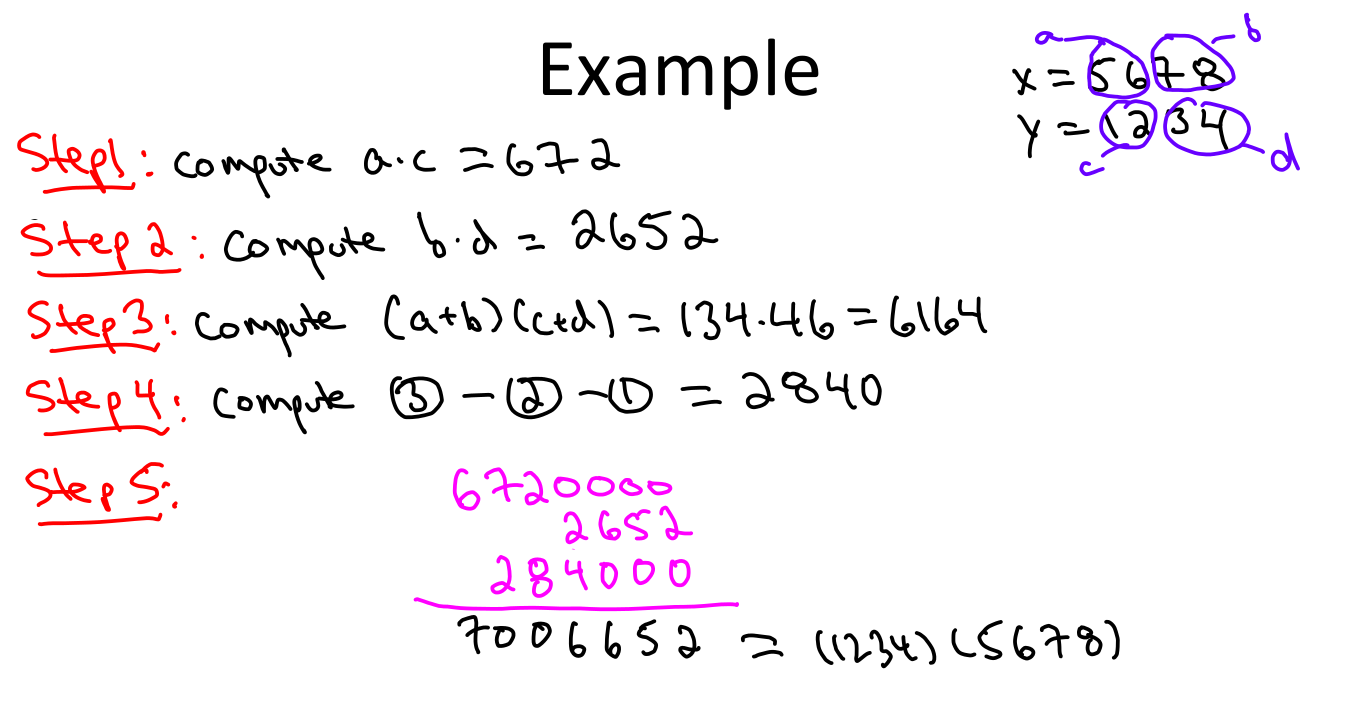U = |A| B| C
V =|D| E| F

PS，这个方法是一开始我的想法，实现后发现只能简化到6次乘法实现，所以最后寻找别的算法。

U  = |U-（m-1）|……|U2 |U1 |U0
V   =|V-（m-1）|……|V2 |V1 |V0

设X = 10^(n/m)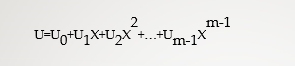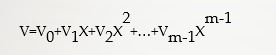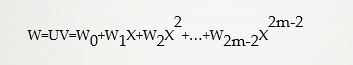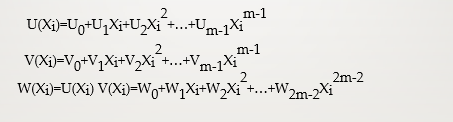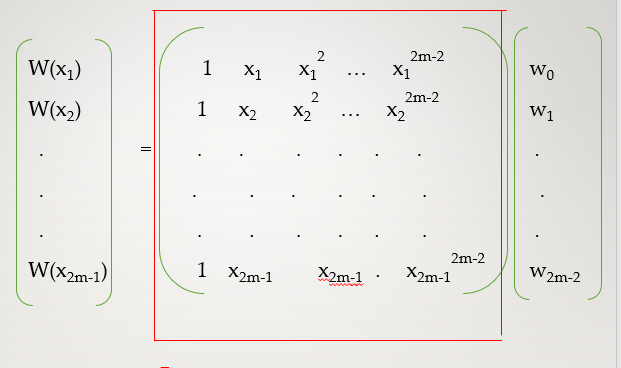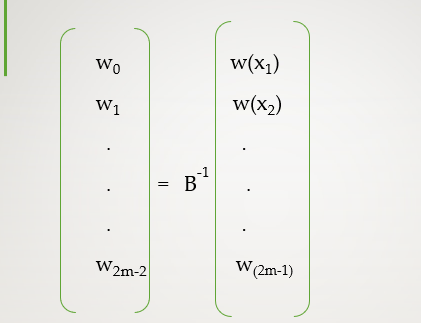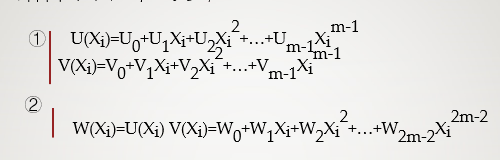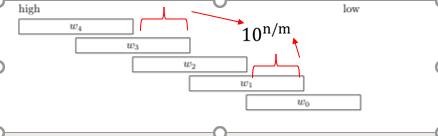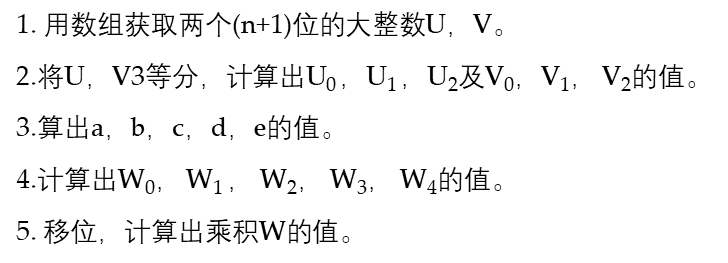U = |U2 |U1 |U0
V =|V2 |V1 |V0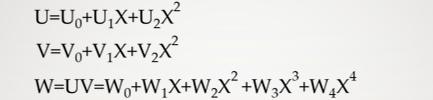X1 = 0，X2 = 1，X3 = -1， X4 = 2，X5 =-2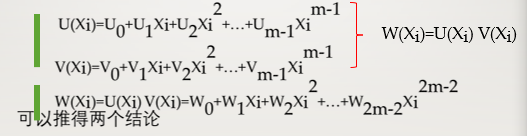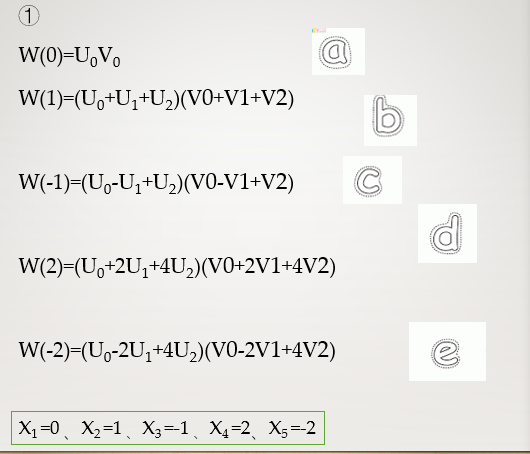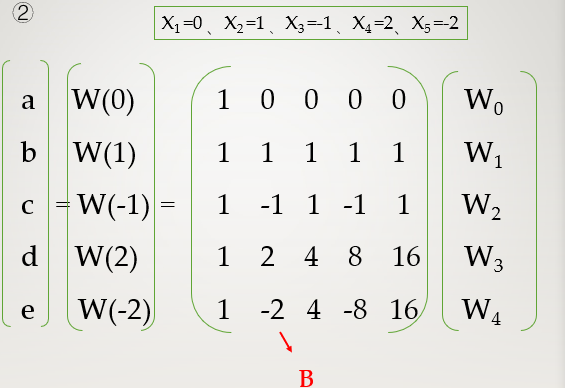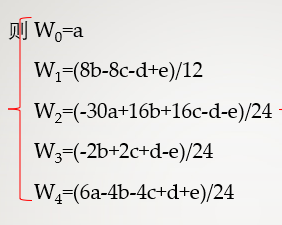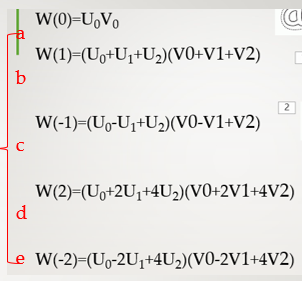123456*987654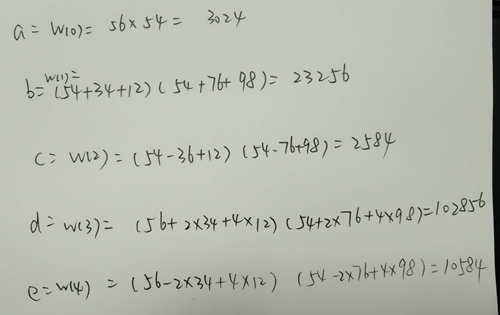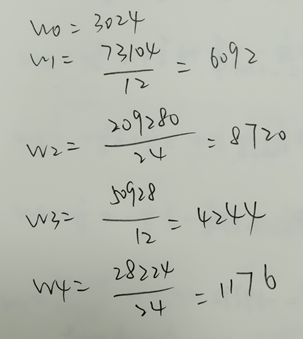UV=W0+W1X+W2X2+W3X3+W4X4 即W0， W1 ， W2， W3， W4移位相加可得W结果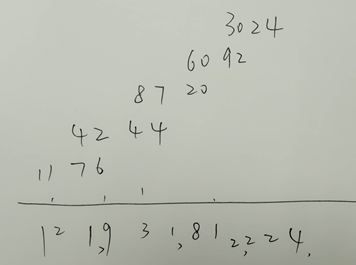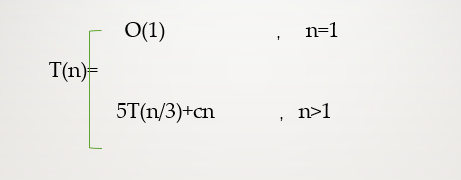TOOM-COOK实现思路和算法可以参考大数乘法问题

/**
* Java8中的 Toom-Cook multiplication 3路乘法
*/
private static BigInteger multiplyToomCook3(BigInteger a, BigInteger b) {
int alen = a.mag.length;
int blen = b.mag.length;

int largest = Math.max(alen, blen);

// k is the size (in ints) of the lower-order slices.
int k = (largest+2)/3;   // Equal to ceil(largest/3)

// r is the size (in ints) of the highest-order slice.
int r = largest - 2*k;

// Obtain slices of the numbers. a2 and b2 are the most significant
// bits of the numbers a and b, and a0 and b0 the least significant.
BigInteger a0, a1, a2, b0, b1, b2;
a2 = a.getToomSlice(k, r, 0, largest);
a1 = a.getToomSlice(k, r, 1, largest);
a0 = a.getToomSlice(k, r, 2, largest);
b2 = b.getToomSlice(k, r, 0, largest);
b1 = b.getToomSlice(k, r, 1, largest);
b0 = b.getToomSlice(k, r, 2, largest);

BigInteger v0, v1, v2, vm1, vinf, t1, t2, tm1, da1, db1;

v0 = a0.multiply(b0);
vm1 = da1.subtract(a1).multiply(db1.subtract(b1));
v1 = da1.multiply(db1);
vinf = a2.multiply(b2);

// The algorithm requires two divisions by 2 and one by 3.
// All divisions are known to be exact, that is, they do not produce
// remainders, and all results are positive.  The divisions by 2 are
// implemented as right shifts which are relatively efficient, leaving
// only an exact division by 3, which is done by a specialized
// linear-time algorithm.
t2 = v2.subtract(vm1).exactDivideBy3();
tm1 = v1.subtract(vm1).shiftRight(1);
t1 = v1.subtract(v0);
t2 = t2.subtract(t1).shiftRight(1);
t1 = t1.subtract(tm1).subtract(vinf);
t2 = t2.subtract(vinf.shiftLeft(1));
tm1 = tm1.subtract(t2);

// Number of bits to shift left.
int ss = k*32;